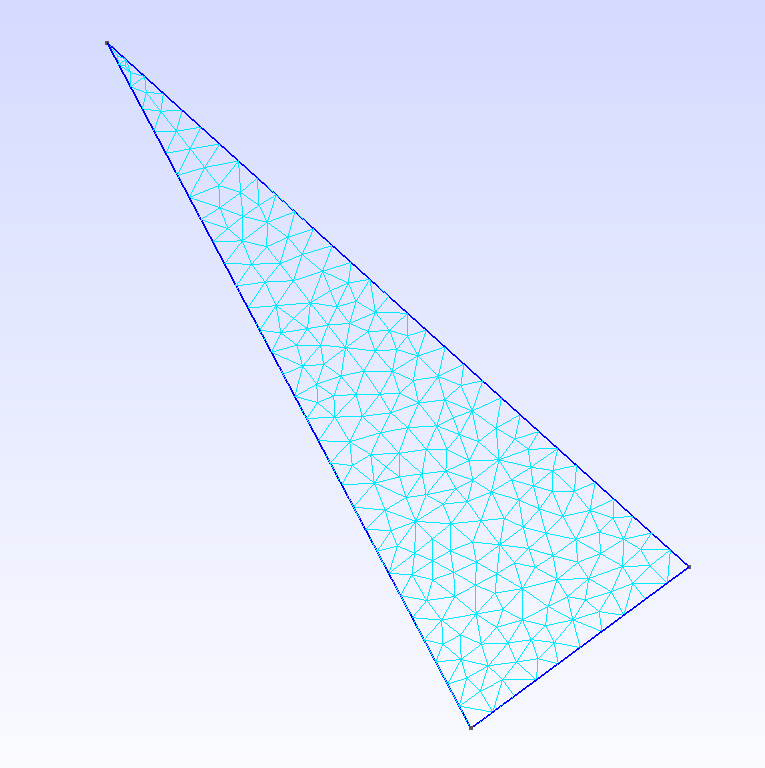# 2D Pendulum

In this example, we simulate the oscillating motion of a highly flexible 2D pendulum.

## 1. Running the case

The command line to run this case is

``mpirun -np 4 feelpp_toolbox_solid --case "github:{repo:toolbox,path:examples/modules/csm/examples/pendulum2D}``
 The report of the execution of the command above is available here.

## 2. Data files

The case data files are available in Github here

## 3. Geometry

The pendulum is an isosceles triangle-shaped mesh as shown below.

Pendulum mesh## 4. Input parameters

### 4.1. Model & Toolbox

We use the Saint-Venant-Kirchhoff hyperelasticity model. We solve for the displacement using a discretization of order 2.

 The model is described in the CSM toolbox documentation, see Computational Solid Mechanics

### 4.2. Materials

We consider a flexible material with the following properties:

Name Description Value Unit

$E_s$

Young’s modulus

$128 \times 10^6$

$kg.m^{-1}.s^{-2}$

$\nu_s$

Poisson’s ration

$0.33$

$dimensionless$

$\rho_s$

density

$8920$

$kg/m^3$

``````  "Materials":
{
"Solid":
{
"E":"128e6", // [kg.m^{-1}.s^{-2}]
"nu":"0.33", // [dimensionless]
"rho":"8920" // [kg/m^3]
}
},``````

We also need gravity:

``````  "Parameters":
{
"gravity":"9.80665" // [m.s^{-2}]
},``````

### 4.3. Volumic force

We also include gravity as a volumic force.

``````"BoundaryConditions":
{
"solid":
{
"displacement":
{
"AxeFixed": // vertex angle -> fixed (no displacement)
{
"expr":"{0,0}"
}
}
}

},``````

### 4.4. Boundary conditions

The pendulum is held by its vertex angle while it oscillates, a zero-displacement condition is set at this vertex. Elsewhere there is a no-stress condition on the boundary.

## 5. Results

The fields of interest are the displacement (in m), the pressure (in Pa), the von Mises yield criterion (dimensionless) and the parallel process id (pid). The pid helps to see how the mesh was partitioned for parallel processing.

``````  "PostProcess":
{
"solid":
{
"Exports":
{
"fields":["displacement","pid","von-mises-criterion"]
},
"Measures":
{
"VolumeVariation":""
}
}
} ``````

### 5.1. Video

The video below shows the oscillating motion of the pendulum.

Oscillation of a 2D triangular pendulum.